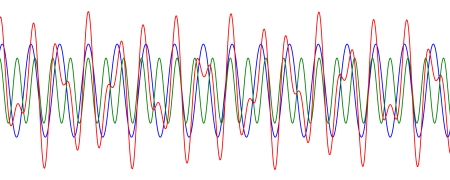# Pretty squiggles

Here’s an image that came out of something I was working on this morning. I thought it might make an interesting border somewhere.The blue line is sin(x), the green line 0.7 sin(φ x), and the red line is their sum. Here φ is the golden ratio (1 + √5)/2. Even though the blue and green curves are both periodic, their sum is not because the ratio of their frequencies is irrational. So you could make this image as long as you’d like and the red curve would never exactly repeat.

Update: See Almost periodic functions

## 11 thoughts on “Pretty squiggles”

1. Little known fact: If you play the red curve through speakers you will hear the voice of God

2. I. J. Kennedy

Very interesting although I can’t say the “border” appeals to me aesthetically.
A few other perhaps surprising facts about sums of periodic functions:

(a) The sum of two periodic functions can be a periodic function with a frequency higher than both constituents.

(b) It is possible to write the function f(x) = x as the sum of two periodic functions.

(c) It is impossible to write x² as the sum of two periodic functions.

3. @IJ (a) seems straight forward but (b) is blowing my mind a little. I assume the periodic functions aren’t continuous?

4. I. J. Kennedy

@Steven, that’s right. In fact, the functions are so strange you need the Axiom of Choice to assert their existence.

5. Do you have a reference? It seems to me any periodic function defined everywhere must be bounded, and the sum of two bounded functions must be bounded… What am I missing?